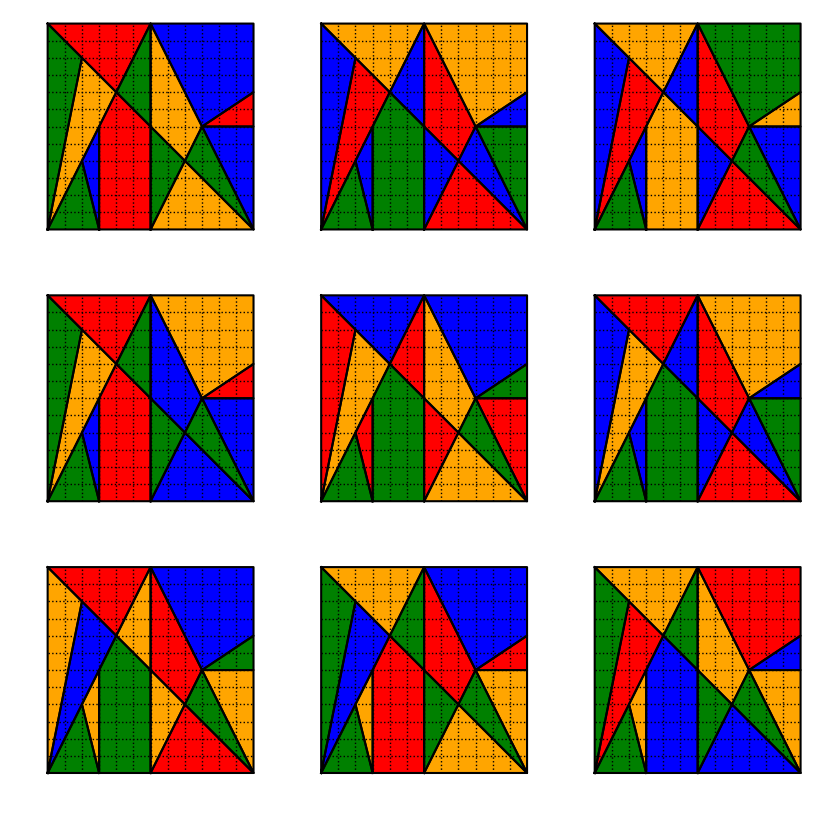## Ostomachion coloring¶

By Laurent Lessard

This problem appeard in The Riddler on January 26, 2018. A full write-up of my solution appears in this blog post.

In :
using JuMP, PyPlot, Gurobi, Combinatorics

In :
# shape coordinates (matrices where first row is xcoords, second row is ycoords)
shapes = [ [0 3 2 0; 0 0 4 0], [3 3 2 3; 0 6 4 0], [3 6 6 4 3 3; 0 0 6 8 6 0], [6 8 6 6; 0 4 6 0],
[6 12 8 6; 0 0 4 0], [0 4 2 0; 0 8 10 0], [0 2 0 0; 0 10 12 0], [0 4 6 0; 12 8 12 12],
[4 6 6 4; 8 6 12 8], [6 8 9 6 6; 6 4 6 12 6], [8 12 9 8; 4 0 6 4], [9 12 12 9; 6 0 6 6],
[9 12 12 9; 6 6 8 6], [9 12 12 6 9; 6 8 12 12 6] ]

# number of shapes in total
N = length(shapes)

# compute areas for each of the shapes
areas = zeros(N,1)
for (i,s) in enumerate(shapes)
x = s[1,:]
y = s[2,:]
k = length(x)
areas[i] = 0.5*(dot(x[1:k-1],y[2:k]) - dot(y[1:k-1],x[2:k]))
end

# specification of which shapes touch which other shapes (edges in the graph)
edges = [(1,2),(1,6),(2,3),(2,6),(3,4),(3,6),(3,9),(4,5),(4,10),
(5,11),(6,7),(6,8),(7,8),(8,9),(9,10),(10,11),(10,14),(11,12),(12,13),(13,14)]

# number of edges
E = length(edges);

In :
C = 4  # number of colors

m = Model(solver=GurobiSolver(OutputFlag=0,PoolSearchMode=2,PoolSolutions=100000))
@variable(m, groups[1:C,1:N], Bin)
@constraint(m, csum[i=1:N], sum(groups[:,i])==1 )  # each shape belongs to exactly one group
@constraint(m, rsum[j=1:C], sum(groups[j,i]*areas[i] for i = 1:N) == sum(areas)/C ) # each group has same frac of tot area
for k = 1:E
@constraint(m, groups[:,edges[k]] + groups[:,edges[k]] .<= 1)  # colors must be different across edges
end
@constraint(m, groups[1,1] == 1)  # the first triangle is green
status = @time solve(m)

Academic license - for non-commercial use only
0.032049 seconds (171 allocations: 53.047 KiB)

Out:
:Optimal
In :
# extract all solutions
g = getrawsolver(m)
solcount = Gurobi.get_sol_count(g)
sols = Dict()
for solnumber = 1:solcount
Gurobi.set_int_param!(g, "SolutionNumber", solnumber-1)
sol = Gurobi.get_dblattrarray(g, "Xn", 1, Gurobi.num_vars(g))
rsol = round.( Int, reshape(sol,(N,C))' )
sols[rsol] = 0
end

# remove duplicate solutions
qq = permutations(collect(1:C))
hh = copy(sols)
for k in keys(hh)
for q in qq
delete!(sols,k[q,:])
end
sols[k] = 0
end
end
println("There are ", length(keys(sols)), " distinct solutions")

There are 9 distinct solutions

In :
# colors for the different regions
col = ["green","blue","red","orange","yellow","cyan"]

# plot the solutions
figure(figsize=(10,10))
for (p,g) in enumerate(keys(sols))
subplot(3,3,p)

# plot each of the shapes
for j = 1:C
gx = find(g[j,:])
for i in gx
fill( shapes[i][1,:], shapes[i][2,:], col[j])
plot( shapes[i][1,:], shapes[i][2,:], "k-")
end
end
axis("image")
axis("off")
# plot the gridlines
for i = 1:11
plot( [i,i], [0,12], ":", linewidth=1, color="black" )
plot( [0,12], [i,i], ":", linewidth=1, color="black" )
end
end
#savefig("osto4.png")In [ ]: